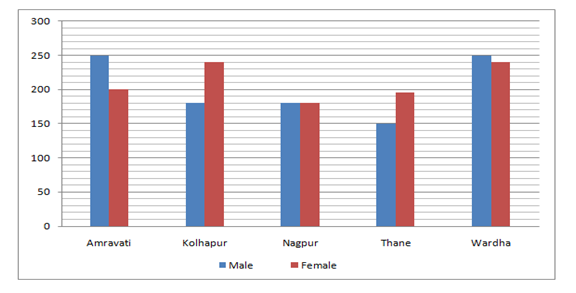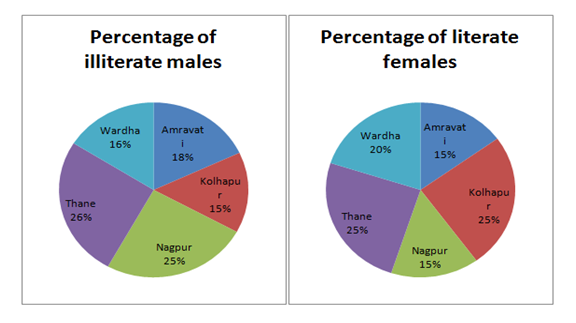# NIACL AO Prelims – Quantitative Aptitude Questions Day- 84

Dear Readers, Bank Exam Race for the Year 2019 is already started, To enrich your preparation here we have providing new series of Practice Questions on Quantitative Aptitude – Section. Candidates those who are preparing for NIACL AO Prelims 2019 Exams can practice these questions daily and make your preparation effective.

[WpProQuiz 4980]

Directions (1 – 5): Following graph shows the number of males and females in five different districts in Maharashtra.1) What is the total number of illiterate females in Nagpur District?

a) 150

b) 163

c) 143

d) 153

e) 133

2) The illiterate females in Amravati District is what per cent more than that in Thane District (take number of girls in thane=196)?

a) 11.8%

b) 15.6%

c) 12.8%

d) 17.2%

e) 16.9%

3) What is the total number of literate males?

a) 714

b) 906

c) 844

d) 794

e) 814

4) The literate females in Kolhapur District is what per cent more than the illiterate males in Nagpur District?

a) 33%

b) 66%

c) 33%

d) 22%

e) 33%

5) What is the total number of illiterate females (take number of girls in thane=196)?

a) 802

b) 838

c) 842

d) 876

e) 854

Directions (6 – 10): What value should come in place of question mark (?) in the following questions?

6) (17)5.6 × (1/4913) ÷ (289)-2.1 = (√17)?

a) 11.2

b) 13.6

c) 9.8

d) 8.4

e) None of these

7) 1475 ÷ 25 + 102 × 98 – (27)2 =?

a) 8754

b) 7365

c) 6892

d) 9326

e) None of these

8) 13824 – 24389 % of 290 + 373248 % of 750 =?

a) 578.3

b) 627.5

c) 479.9

d) 715.1

e) None of these

9) 7 (1/7) – 8 (3/5) + 2 (1/3) – 4 (2/5) = x – 6 (2/3)

a) 4 (2/9)

b) 3 (1/7)

c) 5 (1/9)

d) 6 (3/7)

e) None of these

10) 200 × 75 – 32 × 63 + 12 × 169 = x – 27

a) 15039

b) 18364

c) 17257

d) 22453

e) None of these

Direction (1-5) :

The number of illiterate females of Nagpur District

=180 × 85 / 100 = 153

Illiterate females in Amravati District = 200 × 85 / 100 = 170

Illiterate females in Thane District = 196 × 75 / 100 = 147

Required % = (170 –147) / 147 × 100

= 15.6%

Total number of literate males

= {(250 × 82 / 100) + (180 × 85 / 100) + (180 × 75 / 100) + (150 × 74 / 100) + (250 × 84 / 100)}

= 205 + 153 + 135 + 111 + 210

= 814

The number of literate females of Kolhapur District = 240 × 25 / 100 = 60

The number of illiterate males of Nagpur District = 180 × 25 / 100 = 45

Required % = (60 – 45) / 45 × 100

= 15 / 45 × 100

= 33.33%

Total number of illiterate females of all districts together

= {(200 × 85 / 100) + (240 × 75 / 100) + (180 × 85 / 100) + (196 × 75 / 100) + (240 × 80 / 100)}

= 170 + 180 + 153 + 147 + 192

= 842

Direction  (6-10) :

(√17)11.2 × (√17)-6 ÷ (√17)-8.4 = (√17)?

(√17)11.2 – 6 + 8.4 = (√17)x

(√17)13.6 = (√17)x

X = 13.6

(1475/25) + 102 × 98 – (27)2 = x

X = 59 + 9996 – 729

X = 9326

∛13824 – ∛24389 % of 290 + ∛373248 % of 750 =?

24 – (29 % of 290) + (72 % of 750) = x

24 – (29/100)*290 + (72/100)*750 = x

X = 24 – 84.1 + 540

X = 479.9

7 (1/7) – 8 (3/5) + 2 (1/3) – 4 (2/5) = x – 6 (2/3)

(7- 8 + 2 – 4 + 6)(1/7 – 3/5 + 1/3 – 2/5 + 2/3) = x

X = 3 [1/7 -1+1]

X = 3 (1/7)

200 × 75 – 32 × 63 + 12 × 169 = x – 27

15000 – 2016 + 2028 + 27 = x

X = 17055 – 2016

X = 15039